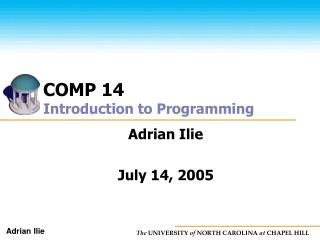DownloadDownload PresentationCOMP 14 Introduction to Programming

# COMP 14 Introduction to Programming

Télécharger la présentation## COMP 14 Introduction to Programming

- - - - - - - - - - - - - - - - - - - - - - - - - - - E N D - - - - - - - - - - - - - - - - - - - - - - - - - - -
##### Presentation Transcript

1. COMP 14Introduction to Programming Adrian Ilie July 14, 2005

2. Arrays • An array is a list of values that can be represented by one variable • Members of an array must all have the same data type • Each value is stored at a specific, numbered position in the array • the number corresponding to each position is called an index or subscript • All arrays have a length • number of elements the array can hold

3. Declaring Arrays The array (element) data type Empty square brackets type[]name; The array (variable) name Creates a reference variable called name that can point to an array of type elements.

4. counter characterSet grade Declaring ArraysExamples // array of counters (integers) int[] counter; // array of characters char[] characterSet; // array of grades (doubles) double[] grade;

5. Instantiating Arrays You must instantiate (create) arrays • the size of an array is typically not known before run time The assignment operator The array (variable) name The new operator name= newtype[size]; The array (element) data type The number of elements

6. counter 0 1 2 3 4 Instantiating ArraysExamples // instantiate an array of counters counter = new int; 0 <= index < size // instantiate the array of grades numStudents = 10; grade = new double[numStudents];

7. Declaration Instantiation Declaration and Instantiation type[]name= new type[size];

8. Example int[] num = new int;

9. Array AccessExamples double score[] = new score; score = 98.3; score = 57.8; score = 93.4; averageScore = (score+score+score)/3; numStudents = 3; totalScore = 0; for (int i = 0; i < numStudents; i++) { totalScore += score[i]; } averageScore = totalScore/numStudents; often use loops for access

10. Array Length Arrays have length • an internal variable called length • number of elements in array • access the length variable using the “dot’ notation (arrayname.length) // loop through the array of test scores sumOfScores = 0; for (int i=0; i<scores.length; i++) { sumOfScores += scores[i]; }

11. Initializing Arrays • Array elements are variables too! • if you don’t initialize, the contents are undefined • When and how? • if you don’t yet know the size • initialize at run time, typically with a loop • if you know how many elements • perhaps use an initializer list int counter[] = {0, 0, 0, 0, 0}; char[] characterSet = {‘a’,’b’,’c’}; // etc.

12. Initializer Lists • List the initial value for the elements of an array • Items are separated by commas and the list is in braces {} • The size of the array is determined by the number of items in the list int[] scores = {87, 98, 45}; • Can only be used in the same statement as declaring the array NOT int[] scores; scores = {87, 98, 45};

13. Array Bounds • Arrays have finite size • If you access an element outside of the array, you’ll get an ArrayIndexOutOfBounds Exception Example: int grades[] = {99, 98, 95, 96}; System.out.println (grades);

14. Example Specify Array Size During Program Execution (Assume thatkeyboard has already been declared and instantiated.) intarraySize; System.out.print ("Enter the size of the array:"); arraySize = Integer.parseInt(keyboard.readLine()); int[] list =new int[arraySize];

15. Example Initialize Array to Specific Value (10.00) (Assume that sale has already been declared and instantiated.) for (int ind = 0; ind < sale.length; ind++) { sale[ind] = 10.00; }

16. Example Read Data into Array (Assume that sale has already been declared and instantiated, and thatkeyboard has already been declared and instantiated.) for (int ind = 0; ind < sale.length; ind++) { sale[ind] = Double.parseDouble(keyboard.readLine()); }

17. Example Print Array (Assume that sale has already been declared and instantiated.) for (int ind = 0; ind < sale.length; ind++) { System.out.print(sale[ind] + " "); }

18. Parallel Arrays Arrays are parallel if corresponding components hold related information String[] studentName; double[] studentGPA; For example, studentName and studentGPA are parallel if studentGPA is the GPA of the student with studentName.

19. In-Class Exercises • Declare an array of integers called numbers • Declare and instantiate an array of 26 characters called alphabet

20. In-Class Exercises • Declare an array of 5 characters called grades and initialize it with the letters: A, B, C, D, F Hint: type[] name = {initialization list}; • Write a loop to print the contents of an array named zipCodes Hint: to access array elementname[index]

21. In-Class Exercises • Write a loop to change all the values of the integer array numbersto index + 1

22. Exercises 1. Find Sum and Average of Array 2. Determine Largest and SmallestElements in Array

23. Homework 6 (practice) • Read data from file • Fill arrays with data • Fill objects with data

24. Tomorrow • More Arrays • arrays of objects • passing arrays as parameters • searching and sorting • Homework 5 due Sunday midnight • Bring laptops!!!